## Chapter IV - Classification

All the questions are as per the ISL seventh printing of the First edition 1.

### Common Stuff

Here I’ll load things I will be using throughout, mostly libraries.

libsUsed<-c("dplyr","ggplot2","tidyverse","ISLR","caret")
invisible(lapply(libsUsed, library, character.only = TRUE))


## Question 4.10 - Page 171

This question should be answered using the Weekly data set, which is part of the ISLR package. This data is similar in nature to the Smarket data from this chapter’s lab, except that it contains 1, 089 weekly returns for 21 years, from the beginning of 1990 to the end of 2010.

(a) Produce some numerical and graphical summaries of the Weekly data. Do there appear to be any patterns?

(b) Use the full data set to perform a logistic regression with Direction as the response and the five lag variables plus Volume as predictors. Use the summary function to print the results. Do any of the predictors appear to be statistically significant? If so, which ones?

(c) Compute the confusion matrix and overall fraction of correct predictions. Explain what the confusion matrix is telling you about the types of mistakes made by logistic regression.

(d) Now fit the logistic regression model using a training data period from 1990 to 2008, with Lag2 as the only predictor. Compute the confusion matrix and the overall fraction of correct predictions for the held out data (that is, the data from 2009 and 2010).

(e) Repeat (d) using LDA.

(f) Repeat (d) using QDA.

(g) Repeat (d) using KNN with $$K = 1$$.

(h) Which of these methods appears to provide the best results on this data?

(i) Experiment with different combinations of predictors, including possible transformations and interactions, for each of the methods. Report the variables, method, and associated confusion matrix that appears to provide the best results on the held out data. Note that you should also experiment with values for K in the KNN classifier.

We will need the data in a variable for ease of use.

weeklyDat<-ISLR::Weekly


### a) Summary Statistics

#### Text

Most of this segment relies heavily on usage of dplyr and especially the %>% or pipe operator for readability. The use of the skimr package2 might added more descriptive statistics, but is not covered here.

#### Basic Summaries

weeklyDat %>% str

# 'data.frame': 1089 obs. of  9 variables:
#  $Year : num 1990 1990 1990 1990 1990 1990 1990 1990 1990 1990 ... #$ Lag1     : num  0.816 -0.27 -2.576 3.514 0.712 ...
#  $Lag2 : num 1.572 0.816 -0.27 -2.576 3.514 ... #$ Lag3     : num  -3.936 1.572 0.816 -0.27 -2.576 ...
#  $Lag4 : num -0.229 -3.936 1.572 0.816 -0.27 ... #$ Lag5     : num  -3.484 -0.229 -3.936 1.572 0.816 ...
#  $Volume : num 0.155 0.149 0.16 0.162 0.154 ... #$ Today    : num  -0.27 -2.576 3.514 0.712 1.178 ...
#  $Direction: Factor w/ 2 levels "Down","Up": 1 1 2 2 2 1 2 2 2 1 ...  We see that there is only one Factor, which makes sense. weeklyDat %>% summary  # Year Lag1 Lag2 Lag3 # Min. :1990 Min. :-18.1950 Min. :-18.1950 Min. :-18.1950 # 1st Qu.:1995 1st Qu.: -1.1540 1st Qu.: -1.1540 1st Qu.: -1.1580 # Median :2000 Median : 0.2410 Median : 0.2410 Median : 0.2410 # Mean :2000 Mean : 0.1506 Mean : 0.1511 Mean : 0.1472 # 3rd Qu.:2005 3rd Qu.: 1.4050 3rd Qu.: 1.4090 3rd Qu.: 1.4090 # Max. :2010 Max. : 12.0260 Max. : 12.0260 Max. : 12.0260 # Lag4 Lag5 Volume Today # Min. :-18.1950 Min. :-18.1950 Min. :0.08747 Min. :-18.1950 # 1st Qu.: -1.1580 1st Qu.: -1.1660 1st Qu.:0.33202 1st Qu.: -1.1540 # Median : 0.2380 Median : 0.2340 Median :1.00268 Median : 0.2410 # Mean : 0.1458 Mean : 0.1399 Mean :1.57462 Mean : 0.1499 # 3rd Qu.: 1.4090 3rd Qu.: 1.4050 3rd Qu.:2.05373 3rd Qu.: 1.4050 # Max. : 12.0260 Max. : 12.0260 Max. :9.32821 Max. : 12.0260 # Direction # Down:484 # Up :605 # # # #  #### Unique Values We might also want to know how many unique values are there in each column. weeklyDat %>% sapply(unique) %>% sapply(length)  # Year Lag1 Lag2 Lag3 Lag4 Lag5 Volume Today # 21 1004 1005 1005 1005 1005 1089 1003 # Direction # 2  We note that year has disproportionately lower values, something to keep in mind while constructing models later. #### Range The range of each variable might be useful as well, but we have to ignore the factor. weeklyDat %>% subset(select=-c(Direction)) %>% sapply(range)  # Year Lag1 Lag2 Lag3 Lag4 Lag5 Volume Today # [1,] 1990 -18.195 -18.195 -18.195 -18.195 -18.195 0.087465 -18.195 # [2,] 2010 12.026 12.026 12.026 12.026 12.026 9.328214 12.026  The most interesting thing about this is probably that the Lag variables all have the same range, also something to be kept in mind while applying transformations to the variable (if at all). #### Mean and Std. Dev By now we might have a pretty good idea of how this will look, but it is still worth seeing. weeklyDat %>% subset(select=-c(Direction)) %>% sapply(mean)  # Year Lag1 Lag2 Lag3 Lag4 Lag5 # 2000.0486685 0.1505849 0.1510790 0.1472048 0.1458182 0.1398926 # Volume Today # 1.5746176 0.1498990  As expected, the Lag values have almost the same mean, what is a bit interesting though, is that the Today variable has roughly the same mean as the Lag variables. weeklyDat %>% subset(select=-c(Direction)) %>% sapply(sd)  # Year Lag1 Lag2 Lag3 Lag4 Lag5 Volume Today # 6.033182 2.357013 2.357254 2.360502 2.360279 2.361285 1.686636 2.356927  This is largely redundant in terms of new information. #### Correlations weeklyDat %>% subset(select=-c(Direction)) %>% cor  # Year Lag1 Lag2 Lag3 Lag4 # Year 1.00000000 -0.032289274 -0.03339001 -0.03000649 -0.031127923 # Lag1 -0.03228927 1.000000000 -0.07485305 0.05863568 -0.071273876 # Lag2 -0.03339001 -0.074853051 1.00000000 -0.07572091 0.058381535 # Lag3 -0.03000649 0.058635682 -0.07572091 1.00000000 -0.075395865 # Lag4 -0.03112792 -0.071273876 0.05838153 -0.07539587 1.000000000 # Lag5 -0.03051910 -0.008183096 -0.07249948 0.06065717 -0.075675027 # Volume 0.84194162 -0.064951313 -0.08551314 -0.06928771 -0.061074617 # Today -0.03245989 -0.075031842 0.05916672 -0.07124364 -0.007825873 # Lag5 Volume Today # Year -0.030519101 0.84194162 -0.032459894 # Lag1 -0.008183096 -0.06495131 -0.075031842 # Lag2 -0.072499482 -0.08551314 0.059166717 # Lag3 0.060657175 -0.06928771 -0.071243639 # Lag4 -0.075675027 -0.06107462 -0.007825873 # Lag5 1.000000000 -0.05851741 0.011012698 # Volume -0.058517414 1.00000000 -0.033077783 # Today 0.011012698 -0.03307778 1.000000000  Useful though this is, it is kind of difficult to work with, in this form, so we might as well programmatic-ally remove strongly correlated data instead. # Uses caret corrCols=weeklyDat %>% subset(select=-c(Direction)) %>% cor %>% findCorrelation(cutoff=0.8) reducedDat<-weeklyDat[-c(corrCols)] reducedDat %>% summary  # Year Lag1 Lag2 Lag3 # Min. :1990 Min. :-18.1950 Min. :-18.1950 Min. :-18.1950 # 1st Qu.:1995 1st Qu.: -1.1540 1st Qu.: -1.1540 1st Qu.: -1.1580 # Median :2000 Median : 0.2410 Median : 0.2410 Median : 0.2410 # Mean :2000 Mean : 0.1506 Mean : 0.1511 Mean : 0.1472 # 3rd Qu.:2005 3rd Qu.: 1.4050 3rd Qu.: 1.4090 3rd Qu.: 1.4090 # Max. :2010 Max. : 12.0260 Max. : 12.0260 Max. : 12.0260 # Lag4 Lag5 Today Direction # Min. :-18.1950 Min. :-18.1950 Min. :-18.1950 Down:484 # 1st Qu.: -1.1580 1st Qu.: -1.1660 1st Qu.: -1.1540 Up :605 # Median : 0.2380 Median : 0.2340 Median : 0.2410 # Mean : 0.1458 Mean : 0.1399 Mean : 0.1499 # 3rd Qu.: 1.4090 3rd Qu.: 1.4050 3rd Qu.: 1.4050 # Max. : 12.0260 Max. : 12.0260 Max. : 12.0260  We can see that the Volume variable has been dropped, since it evidently is strongly correlated with Year. This may or may not be a useful insight, but it is good to keep in mind. #### Visualization We will be using the ggplot2 library throughout for this segment. Lets start with some scatter plots in a one v/s all scheme, similar to the methodology described here. weeklyDat %>% subset(select=-c(Direction)) %>% gather(-Year,key="Variable", value="Value") %>% ggplot(aes(x=Value,y=Year)) + geom_point() + facet_wrap(~Variable) + coord_flip()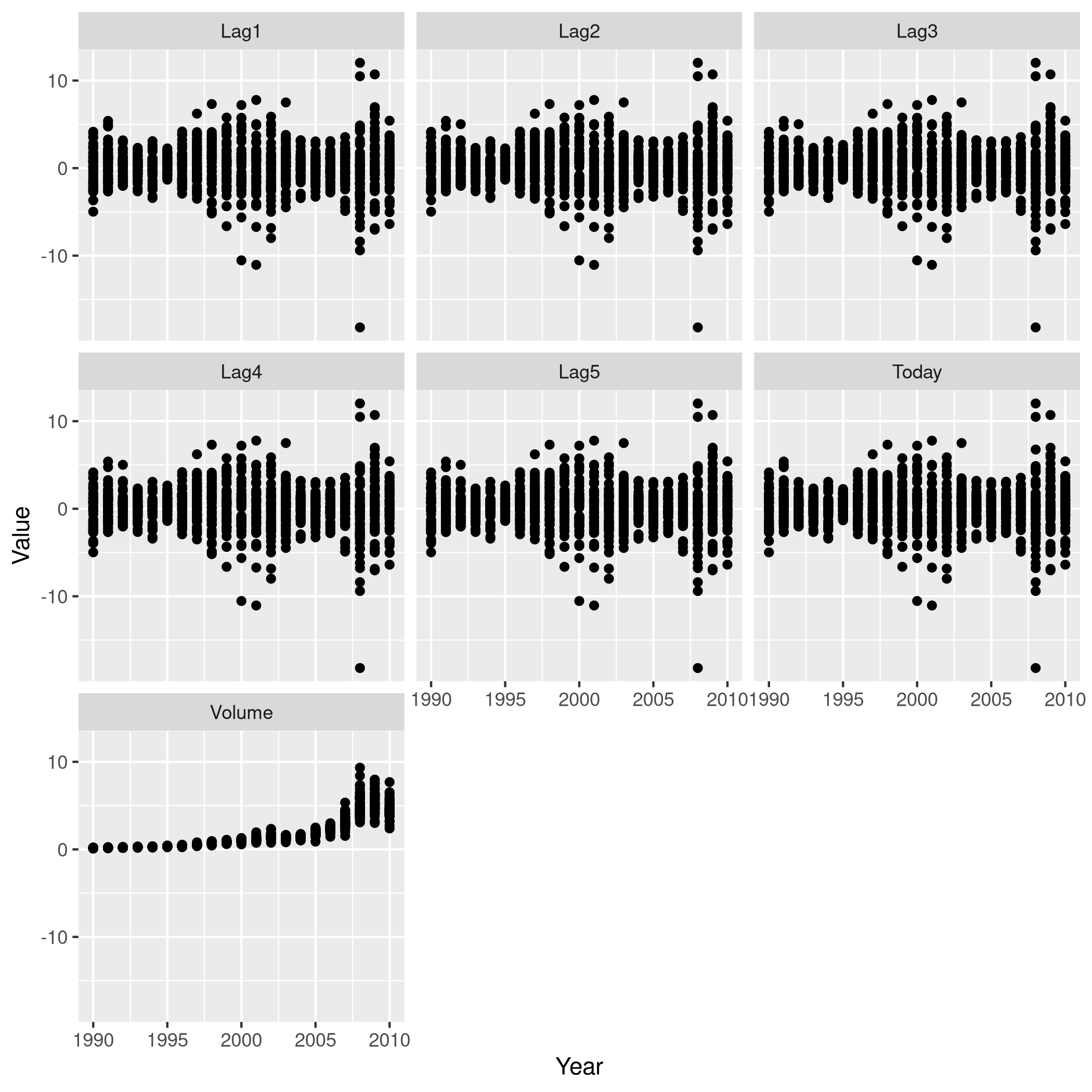Figure 1: One v/s all for Direction That didn’t really tell us much which we didn’t already get from the cor() function, but we can go the whole hog and do this for every variable since we don’t have that many in the first place.. weeklyDat %>% subset(select=-c(Direction)) %>% pairs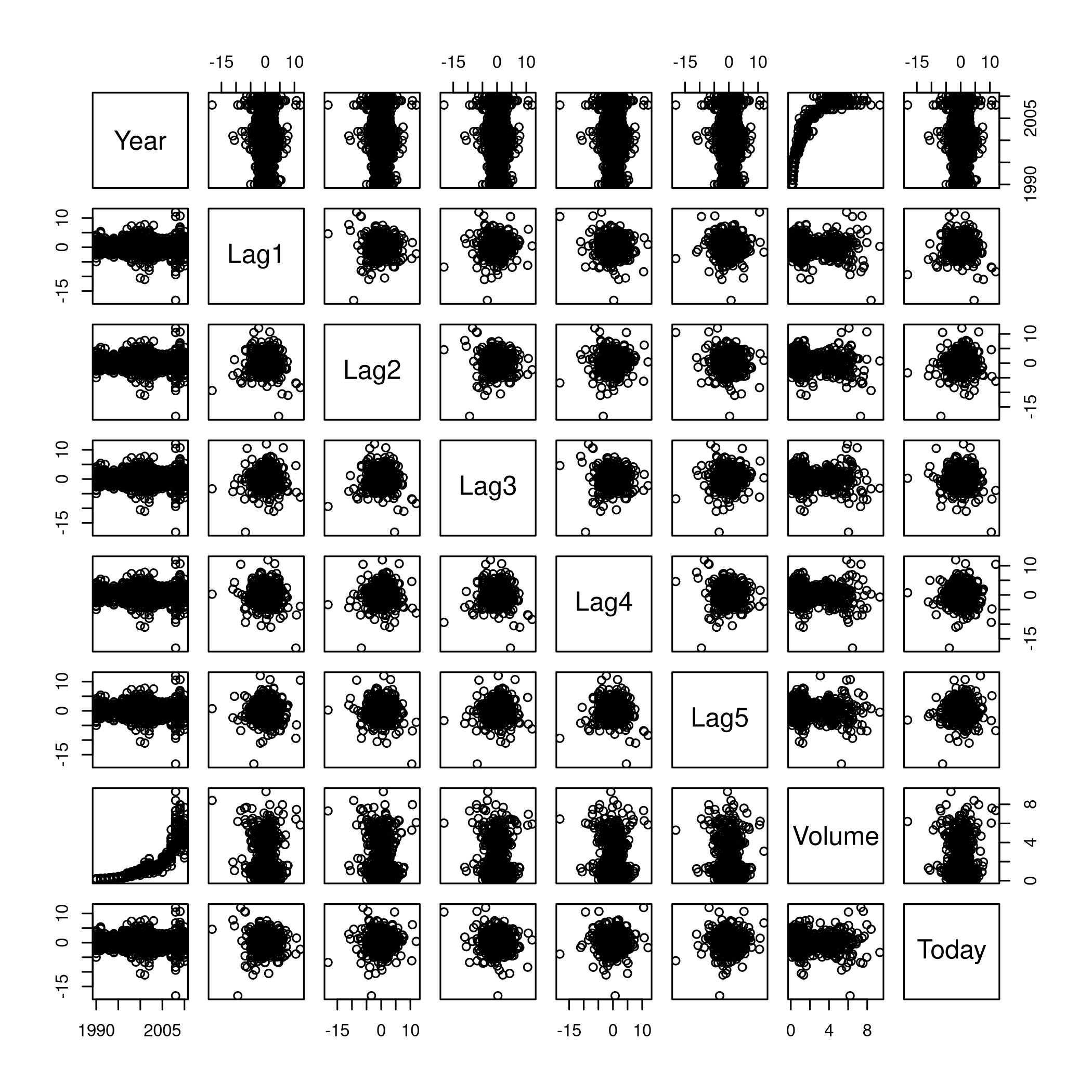Figure 2: Pairs This is not especially useful, and it is doubtful if more scatter-plots will help at all, so lets move on to box plots. weeklyDat %>% pivot_longer(-c(Direction,Volume,Today,Year),names_to="Lag",values_to="Value") %>% ggplot(aes(x=Direction,y=Value,fill=Lag)) + geom_boxplot()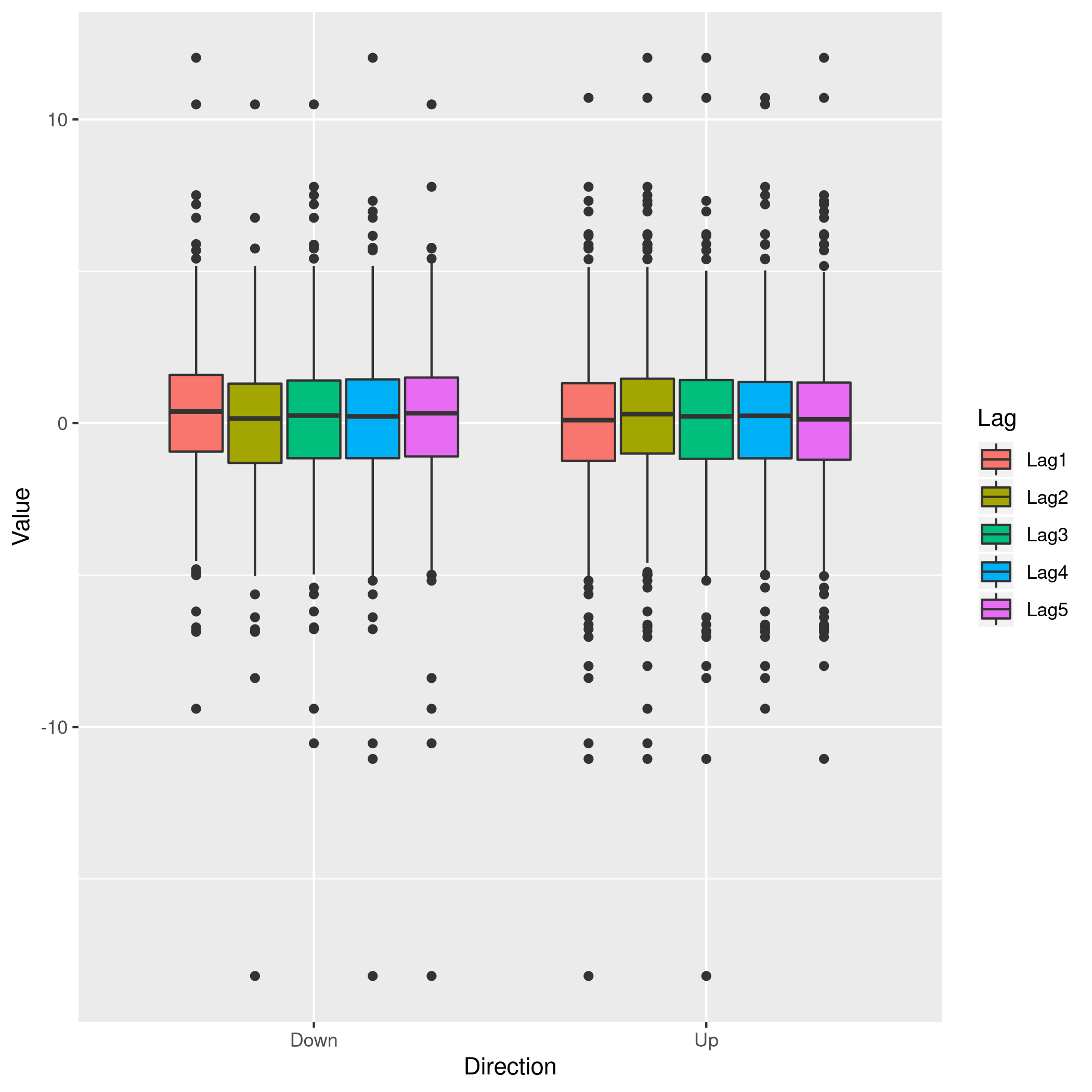Figure 3: Box plots for Direction weeklyDat %>% pivot_longer(-c(Direction,Volume,Today,Year),names_to="Lag",values_to="Value") %>% ggplot(aes(x=Today,y=Value,fill=Lag)) + geom_boxplot()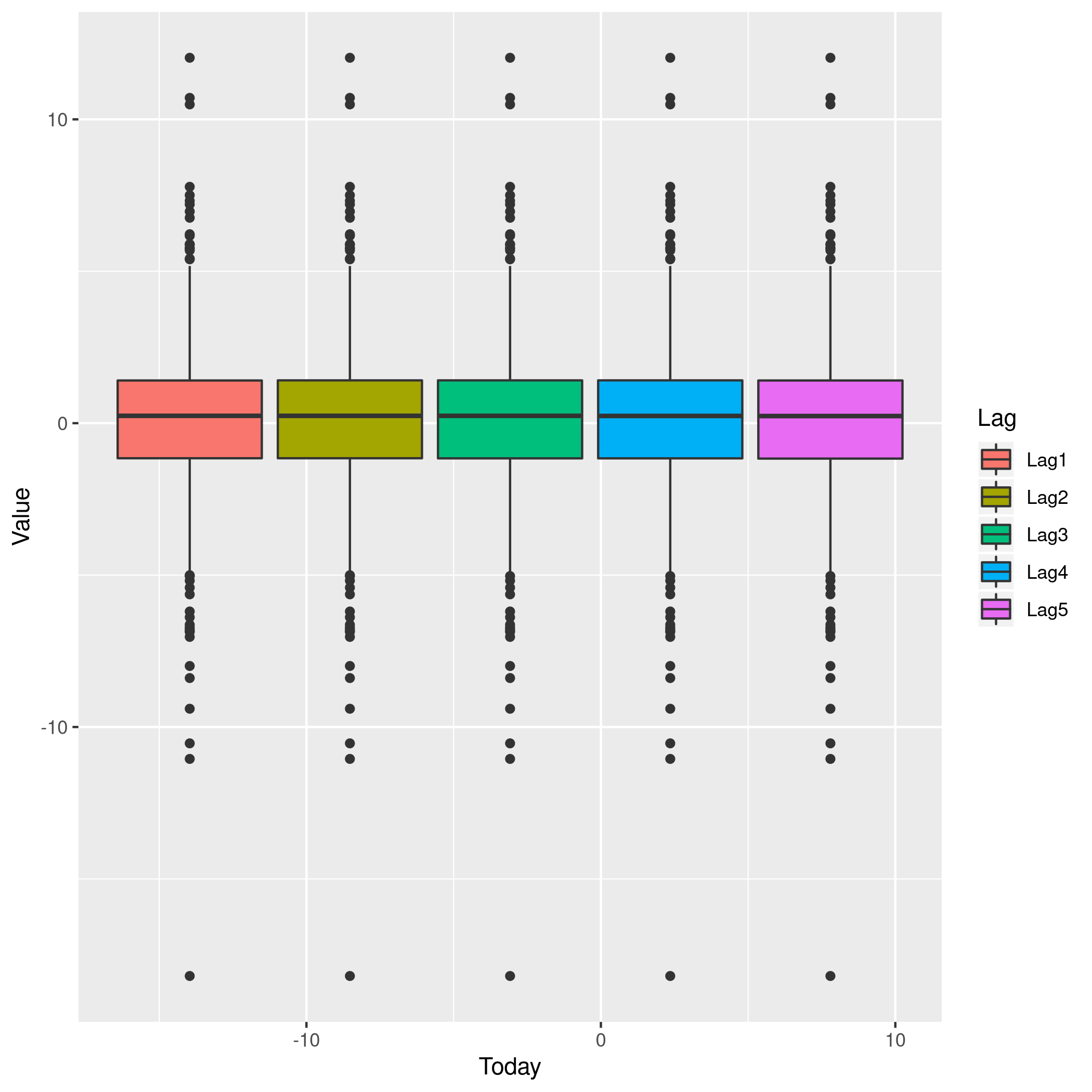Figure 4: More box plots weeklyDat %>% pivot_longer(-c(Direction,Volume,Today,Year),names_to="Lag",values_to="Value") %>% ggplot(aes(x=Lag,y=Value,fill=Direction)) + geom_boxplot()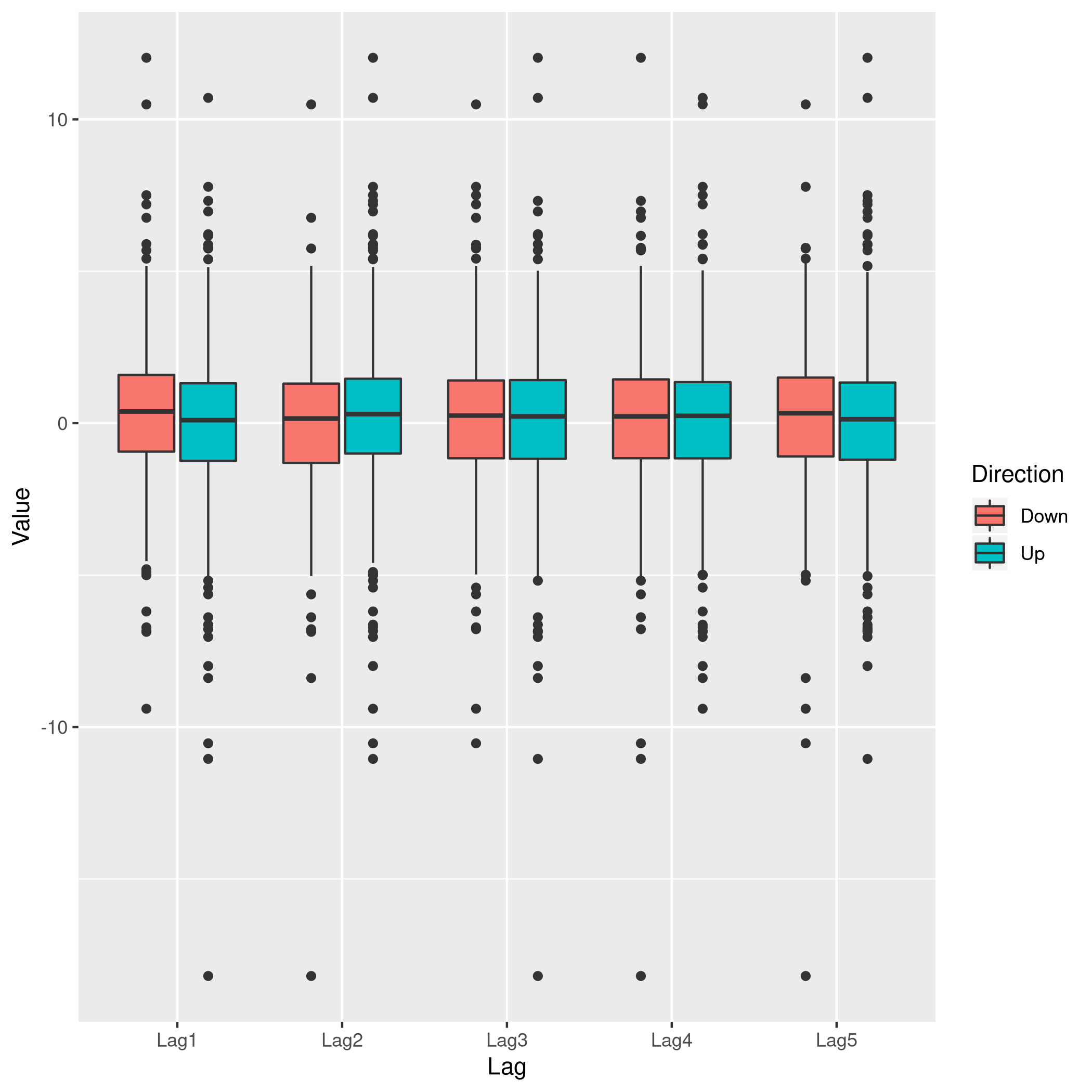Figure 5: Lag v/s all This does summarize our text analysis quite well. Importantly, it tells us that the Today value is largely unrelated to the $$4$$ Lag variables. A really good-looking box-plot is easy to get with the caret library: weeklyDat %>% subset(select=-c(Direction)) %>% featurePlot( y = weeklyDat$Direction,
plot = "box",
# Pass in options to bwplot()
scales = list(y = list(relation="free"),
x = list(rot = 90)),
auto.key = list(columns = 2))


We might want to visualize our correlation matrix as well.

library(reshape2)

#
# Attaching package: 'reshape2'

# The following object is masked from 'package:tidyr':
#
#     smiths

weeklyDat %>% subset(select=-c(Direction)) %>% cor %>% melt %>% ggplot(aes(x=Var1,y=Var2,fill=value)) +
geom_tile()


### b) Logistic Regression - Predictor Significance

Lets start with the native glm function.

glm.fit=glm(Direction~Lag1+Lag2+Lag3+Lag4+Lag5+Volume, data=weeklyDat, family=binomial)
summary(glm.fit)

#
# Call:
# glm(formula = Direction ~ Lag1 + Lag2 + Lag3 + Lag4 + Lag5 +
#     Volume, family = binomial, data = weeklyDat)
#
# Deviance Residuals:
#     Min       1Q   Median       3Q      Max
# -1.6949  -1.2565   0.9913   1.0849   1.4579
#
# Coefficients:
#             Estimate Std. Error z value Pr(>|z|)
# (Intercept)  0.26686    0.08593   3.106   0.0019 **
# Lag1        -0.04127    0.02641  -1.563   0.1181
# Lag2         0.05844    0.02686   2.175   0.0296 *
# Lag3        -0.01606    0.02666  -0.602   0.5469
# Lag4        -0.02779    0.02646  -1.050   0.2937
# Lag5        -0.01447    0.02638  -0.549   0.5833
# Volume      -0.02274    0.03690  -0.616   0.5377
# ---
# Signif. codes:  0 '***' 0.001 '**' 0.01 '*' 0.05 '.' 0.1 ' ' 1
#
# (Dispersion parameter for binomial family taken to be 1)
#
#     Null deviance: 1496.2  on 1088  degrees of freedom
# Residual deviance: 1486.4  on 1082  degrees of freedom
# AIC: 1500.4
#
# Number of Fisher Scoring iterations: 4


Evidently, only the Lag2 value is of statistical significance.

It is always of importance to figure out what numeric values R will assign to our factors, and it is best not to guess.

contrasts(weeklyDat$Direction)  # Up # Down 0 # Up 1  ### c) Confusion Matrix and Metrics Essentially: • Predict the response • Create an output length vector • Apply thresholding to obtain labels glm.probs = predict(glm.fit, type = "response") glm.pred = rep("Up",length(glm.probs)) glm.pred[glm.probs<0.5]="Down" glm.pred=factor(glm.pred) confusionMatrix(glm.pred,weeklyDat$Direction)

# Confusion Matrix and Statistics
#
#           Reference
# Prediction Down  Up
#       Down   54  48
#       Up    430 557
#
#                Accuracy : 0.5611
#                  95% CI : (0.531, 0.5908)
#     No Information Rate : 0.5556
#     P-Value [Acc > NIR] : 0.369
#
#                   Kappa : 0.035
#
#  Mcnemar's Test P-Value : <2e-16
#
#             Sensitivity : 0.11157
#             Specificity : 0.92066
#          Pos Pred Value : 0.52941
#          Neg Pred Value : 0.56434
#              Prevalence : 0.44444
#          Detection Rate : 0.04959
#    Detection Prevalence : 0.09366
#       Balanced Accuracy : 0.51612
#
#        'Positive' Class : Down
#

• We have used the confusionMatrix function from caret (documented here) instead of displaying the results with table and then calculating precision, recall and the rest by hand.

### d) Train Test Splits

Although we could have used the indices and passed it to glm as the subset attribute, it is cleaner to just make subsets instead.

weeklyVal<-weeklyDat %>% filter(Year>=2009)
weeklyTrain<-weeklyDat %>% filter(Year<2009)


Now we can train a model on our training data.

glm.fit=glm(Direction~Lag2,data=weeklyTrain,family=binomial)
summary(glm.fit)

#
# Call:
# glm(formula = Direction ~ Lag2, family = binomial, data = weeklyTrain)
#
# Deviance Residuals:
#    Min      1Q  Median      3Q     Max
# -1.536  -1.264   1.021   1.091   1.368
#
# Coefficients:
#             Estimate Std. Error z value Pr(>|z|)
# (Intercept)  0.20326    0.06428   3.162  0.00157 **
# Lag2         0.05810    0.02870   2.024  0.04298 *
# ---
# Signif. codes:  0 '***' 0.001 '**' 0.01 '*' 0.05 '.' 0.1 ' ' 1
#
# (Dispersion parameter for binomial family taken to be 1)
#
#     Null deviance: 1354.7  on 984  degrees of freedom
# Residual deviance: 1350.5  on 983  degrees of freedom
# AIC: 1354.5
#
# Number of Fisher Scoring iterations: 4


Having fit our model, we will test the predictions on our held out data.

glm.probs = predict(glm.fit,weeklyVal, type = "response")
glm.pred = rep("Up",length(glm.probs))
glm.pred[glm.probs<0.5]="Down"
glm.pred=factor(glm.pred)
confusionMatrix(glm.pred,weeklyVal$Direction)  # Confusion Matrix and Statistics # # Reference # Prediction Down Up # Down 9 5 # Up 34 56 # # Accuracy : 0.625 # 95% CI : (0.5247, 0.718) # No Information Rate : 0.5865 # P-Value [Acc > NIR] : 0.2439 # # Kappa : 0.1414 # # Mcnemar's Test P-Value : 7.34e-06 # # Sensitivity : 0.20930 # Specificity : 0.91803 # Pos Pred Value : 0.64286 # Neg Pred Value : 0.62222 # Prevalence : 0.41346 # Detection Rate : 0.08654 # Detection Prevalence : 0.13462 # Balanced Accuracy : 0.56367 # # 'Positive' Class : Down #  We really aren’t doing very well with this single variable model as is evident. ### e) LDA models At this stage we could use MASS to get the lda function, but it would be better to just switch to using caret. Note that the caret prediction is a label by default, so thresholding needs to be specified differently if required. lda.fit=train(Direction~Lag2,data=weeklyTrain,method="lda") summary(lda.fit)  # Length Class Mode # prior 2 -none- numeric # counts 2 -none- numeric # means 2 -none- numeric # scaling 1 -none- numeric # lev 2 -none- character # svd 1 -none- numeric # N 1 -none- numeric # call 3 -none- call # xNames 1 -none- character # problemType 1 -none- character # tuneValue 1 data.frame list # obsLevels 2 -none- character # param 0 -none- list  predict(lda.fit,weeklyVal) %>% confusionMatrix(weeklyVal$Direction)

# Confusion Matrix and Statistics
#
#           Reference
# Prediction Down Up
#       Down    9  5
#       Up     34 56
#
#                Accuracy : 0.625
#                  95% CI : (0.5247, 0.718)
#     No Information Rate : 0.5865
#     P-Value [Acc > NIR] : 0.2439
#
#                   Kappa : 0.1414
#
#  Mcnemar's Test P-Value : 7.34e-06
#
#             Sensitivity : 0.20930
#             Specificity : 0.91803
#          Pos Pred Value : 0.64286
#          Neg Pred Value : 0.62222
#              Prevalence : 0.41346
#          Detection Rate : 0.08654
#    Detection Prevalence : 0.13462
#       Balanced Accuracy : 0.56367
#
#        'Positive' Class : Down
#


### f) QDA models

qda.fit=train(Direction~Lag2,data=weeklyTrain,method="qda")
summary(qda.fit)

#             Length Class      Mode
# prior       2      -none-     numeric
# counts      2      -none-     numeric
# means       2      -none-     numeric
# scaling     2      -none-     numeric
# ldet        2      -none-     numeric
# lev         2      -none-     character
# N           1      -none-     numeric
# call        3      -none-     call
# xNames      1      -none-     character
# problemType 1      -none-     character
# tuneValue   1      data.frame list
# obsLevels   2      -none-     character
# param       0      -none-     list

confusionMatrix(knn.pred,weeklyVal$Direction)  # Confusion Matrix and Statistics # # Reference # Prediction Down Up # Down 21 30 # Up 22 31 # # Accuracy : 0.5 # 95% CI : (0.4003, 0.5997) # No Information Rate : 0.5865 # P-Value [Acc > NIR] : 0.9700 # # Kappa : -0.0033 # # Mcnemar's Test P-Value : 0.3317 # # Sensitivity : 0.4884 # Specificity : 0.5082 # Pos Pred Value : 0.4118 # Neg Pred Value : 0.5849 # Prevalence : 0.4135 # Detection Rate : 0.2019 # Detection Prevalence : 0.4904 # Balanced Accuracy : 0.4983 # # 'Positive' Class : Down #  Clearly this model is not doing very well. ### h) Model Selection We will first get the ROC curves. library(pROC)  # Type 'citation("pROC")' for a citation.  # # Attaching package: 'pROC'  # The following objects are masked from 'package:stats': # # cov, smooth, var  knnROC<-roc(predictor=as.numeric(knn.pred),response=weeklyVal$Direction,levels=rev(levels(weeklyVal$Direction)))  # Setting direction: controls < cases  logiROC<-roc(predictor=as.numeric(predict(glm.fit,weeklyVal)),response=weeklyVal$Direction)

# Setting levels: control = Down, case = Up

# Setting direction: controls > cases

ldaROC<-roc(predictor=as.numeric(predict(lda.fit,weeklyVal)),response=weeklyVal$Direction)  # Setting levels: control = Down, case = Up  # Setting direction: controls < cases  qdaROC<-roc(predictor=as.numeric(predict(qda.fit,weeklyVal)),response=weeklyVal$Direction)

# Setting levels: control = Down, case = Up
# Setting direction: controls < cases


Now to plot them.

ggroc(list(KNN=knnROC,Logistic=logiROC,LDA=ldaROC,QDA=qdaROC))


To compare models with caret it is easy to refit the logistic and knn models in the caret formulation.

knnCaret=train(Direction~Lag2,data=weeklyTrain,method="knn")


However, the KNN model is the best parameter model.

resmod <- resamples(list(lda=lda.fit, qda=qda.fit, KNN=knnCaret))
summary(resmod)

#
# Call:
# summary.resamples(object = resmod)
#
# Models: lda, qda, KNN
# Number of resamples: 25
#
# Accuracy
#          Min.   1st Qu.    Median      Mean   3rd Qu.      Max. NA's
# lda 0.5043228 0.5344353 0.5529101 0.5500861 0.5683060 0.5846995    0
# qda 0.5044248 0.5204360 0.5307263 0.5326785 0.5462428 0.5777778    0
# KNN 0.4472222 0.5082873 0.5240642 0.5168327 0.5302198 0.5485714    0
#
# Kappa
#            Min.      1st Qu.      Median         Mean    3rd Qu.       Max.
# lda -0.02618939 -0.003638168 0.005796908  0.007801904 0.01635328 0.05431238
# qda -0.06383592 -0.005606123 0.000000000 -0.003229697 0.00000000 0.03606344
# KNN -0.11297539  0.004168597 0.024774647  0.016171229 0.04456142 0.07724439
#     NA's
# lda    0
# qda    0
# KNN    0

bwplot(resmod)

dotplot(resmod)


Kappa or Cohen’s Kappa is essentially classification accuracy, normalized at the baseline of random chance. It is a more useful measure to use on problems that have imbalanced classes. There’s more on model selection here.

### i) Further Tuning

Do note the caret defaults.

fitControl <- trainControl(# 10-fold CV
method = "repeatedcv",
number = 10,
# repeated ten times
repeats = 10)


### Logistic

glm2.fit=glm(Direction~Lag1+Lag2+Lag3+Lag4+Lag5+Volume, data=weeklyDat, family=binomial)

glm2.probs = predict(glm2.fit,weeklyVal, type = "response")
glm2.pred = rep("Up",length(glm2.probs))
glm2.pred[glm2.probs<0.5]="Down"
glm2.pred=factor(glm2.pred)
confusionMatrix(glm2.pred,weeklyVal$Direction)  # Confusion Matrix and Statistics # # Reference # Prediction Down Up # Down 17 13 # Up 26 48 # # Accuracy : 0.625 # 95% CI : (0.5247, 0.718) # No Information Rate : 0.5865 # P-Value [Acc > NIR] : 0.24395 # # Kappa : 0.1907 # # Mcnemar's Test P-Value : 0.05466 # # Sensitivity : 0.3953 # Specificity : 0.7869 # Pos Pred Value : 0.5667 # Neg Pred Value : 0.6486 # Prevalence : 0.4135 # Detection Rate : 0.1635 # Detection Prevalence : 0.2885 # Balanced Accuracy : 0.5911 # # 'Positive' Class : Down #  #### QDA qdaCaret=train(Direction~Lag2+Lag4,data=weeklyTrain,method="qda",trainControl=fitControl)  summary(qdaCaret)  # Length Class Mode # prior 2 -none- numeric # counts 2 -none- numeric # means 4 -none- numeric # scaling 8 -none- numeric # ldet 2 -none- numeric # lev 2 -none- character # N 1 -none- numeric # call 4 -none- call # xNames 2 -none- character # problemType 1 -none- character # tuneValue 1 data.frame list # obsLevels 2 -none- character # param 1 -none- list  predict(qdaCaret,weeklyVal) %>% confusionMatrix(weeklyVal$Direction)

# Confusion Matrix and Statistics
#
#           Reference
# Prediction Down Up
#       Down    9 14
#       Up     34 47
#
#                Accuracy : 0.5385
#                  95% CI : (0.438, 0.6367)
#     No Information Rate : 0.5865
#     P-Value [Acc > NIR] : 0.863079
#
#                   Kappa : -0.0217
#
#  Mcnemar's Test P-Value : 0.006099
#
#             Sensitivity : 0.20930
#             Specificity : 0.77049
#          Pos Pred Value : 0.39130
#          Neg Pred Value : 0.58025
#              Prevalence : 0.41346
#          Detection Rate : 0.08654
#    Detection Prevalence : 0.22115
#       Balanced Accuracy : 0.48990
#
#        'Positive' Class : Down
#


#### LDA

ldaCaret=train(Direction~Lag2+Lag1+Year,data=weeklyTrain,method="lda",trainControl=fitControl)

summary(ldaCaret)

#             Length Class      Mode
# prior       2      -none-     numeric
# counts      2      -none-     numeric
# means       6      -none-     numeric
# scaling     3      -none-     numeric
# lev         2      -none-     character
# svd         1      -none-     numeric
# N           1      -none-     numeric
# call        4      -none-     call
# xNames      3      -none-     character
# problemType 1      -none-     character
# tuneValue   1      data.frame list
# obsLevels   2      -none-     character
# param       1      -none-     list

predict(ldaCaret,weeklyVal) %>% confusionMatrix(weeklyVal$Direction)  # Confusion Matrix and Statistics # # Reference # Prediction Down Up # Down 20 19 # Up 23 42 # # Accuracy : 0.5962 # 95% CI : (0.4954, 0.6913) # No Information Rate : 0.5865 # P-Value [Acc > NIR] : 0.4626 # # Kappa : 0.1558 # # Mcnemar's Test P-Value : 0.6434 # # Sensitivity : 0.4651 # Specificity : 0.6885 # Pos Pred Value : 0.5128 # Neg Pred Value : 0.6462 # Prevalence : 0.4135 # Detection Rate : 0.1923 # Detection Prevalence : 0.3750 # Balanced Accuracy : 0.5768 # # 'Positive' Class : Down #  #### KNN Honestly, again, this should be scaled. Plot KNN with the best parameters. plot(knnCaret)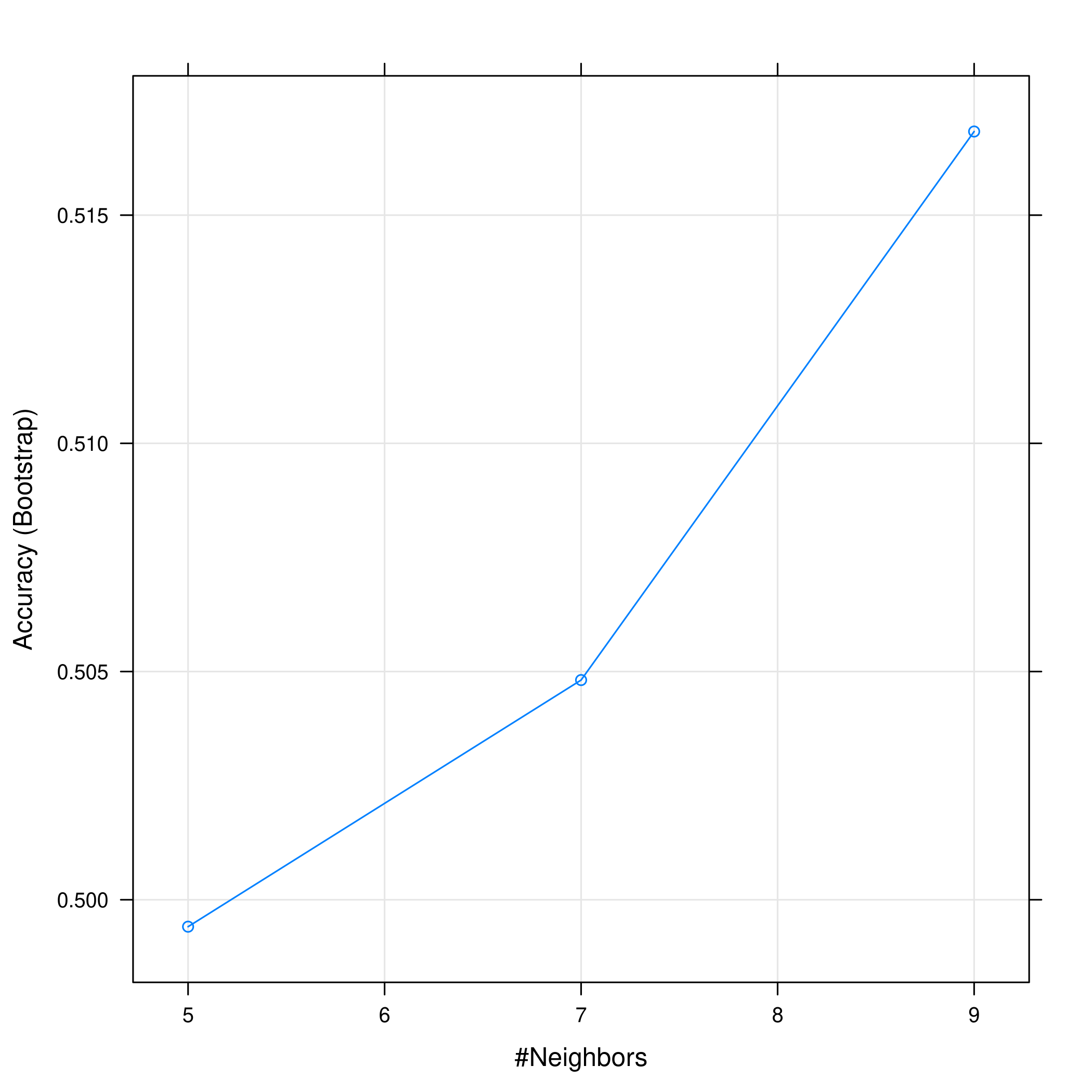Figure 10: KNN statistics Evidently, the accuracy increases with an increase in the number of neighbors considered. plot(knnCaret, print.thres = 0.5, type="S")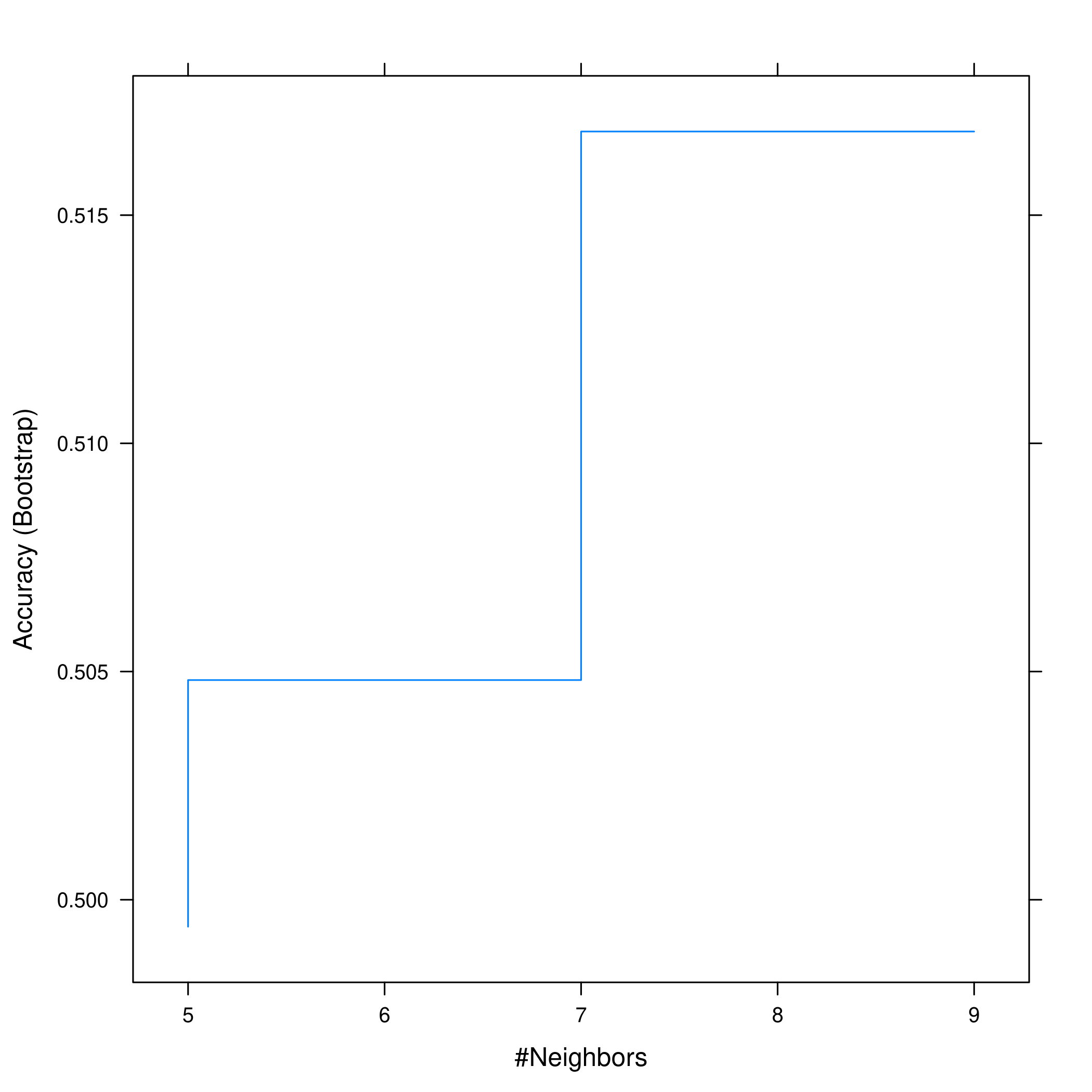Figure 11: Visualizing thresholds for KNN However this shows that we don’t actually get much of an increase in accuracy anyway. ## Question 4.11 - Pages 171-172 In this problem, you will develop a model to predict whether a given car gets high or low gas mileage based on the Auto data set. (a) Create a binary variable, mpg01 , that contains a 1 if mpg contains a value above its median, and a 0 if mpg contains a value below its median. You can compute the median using the median() function. Note you may find it helpful to use the data.frame() function to create a single data set containing both mpg01 and the other Auto variables. (b) Explore the data graphically in order to investigate the association between mpg01 and the other features. Which of the other features seem most likely to be useful in predicting mpg01 ? Scatter-plots and boxplots may be useful tools to answer this question. Describe your findings. (c) Split the data into a training set and a test set. (d) Perform LDA on the training data in order to predict mpg01 using the variables that seemed most associated with mpg01 in (b). What is the test error of the model obtained? (e) Perform QDA on the training data in order to predict mpg01 using the variables that seemed most associated with mpg01 in (b). What is the test error of the model obtained? (f) Perform logistic regression on the training data in order to predict mpg01 using the variables that seemed most associated with mpg01 in (b). What is the test error of the model obtained? (g) Perform KNN on the training data, with several values of $$K$$, in order to predict mpg01 . Use only the variables that seemed most associated with mpg01 in (b). What test errors do you obtain? Which value of $$K$$ seems to perform the best on this data set? ### Answer autoDat<-ISLR::Auto  ### a) Binary Variable autoDat$mpg %>% sort() %>% median()

#  22.75


Now we can get a new variable from that.

newDat=autoDat
newDat$mpg01 <- ifelse(autoDat$mpg<autoDat$mpg %>% sort() %>% median(),0,1) %>% factor()  Note that the ifelse command takes a truthy function, value when false, value when true, but does not return a factor automatically so we piped it to factor to ensure it is factorial. ### b) Visual Exploration Some box-plots: newDat %>% pivot_longer(-c(mpg01,name),names_to="Params",values_to="Value") %>% ggplot(aes(x=mpg01,y=Value)) + geom_boxplot() + facet_wrap(~ Params, scales = "free_y")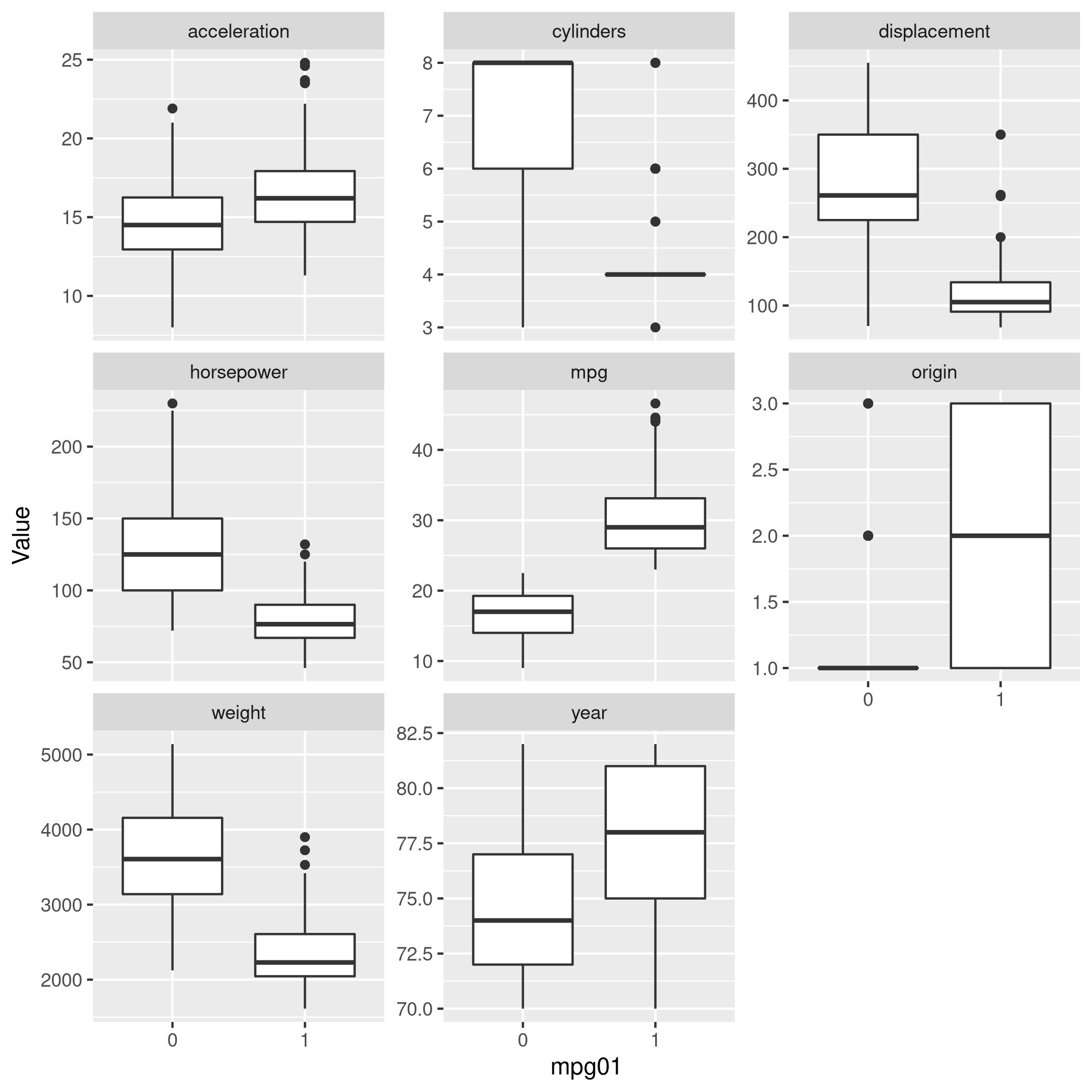Figure 12: Box plots With some scatter plots as well: newDat %>% pivot_longer(-c(mpg01,name,weight),names_to="Params",values_to="Value") %>% ggplot(aes(x=weight,y=Value,color=mpg01)) + geom_point() + facet_wrap(~ Params, scales = "free_y")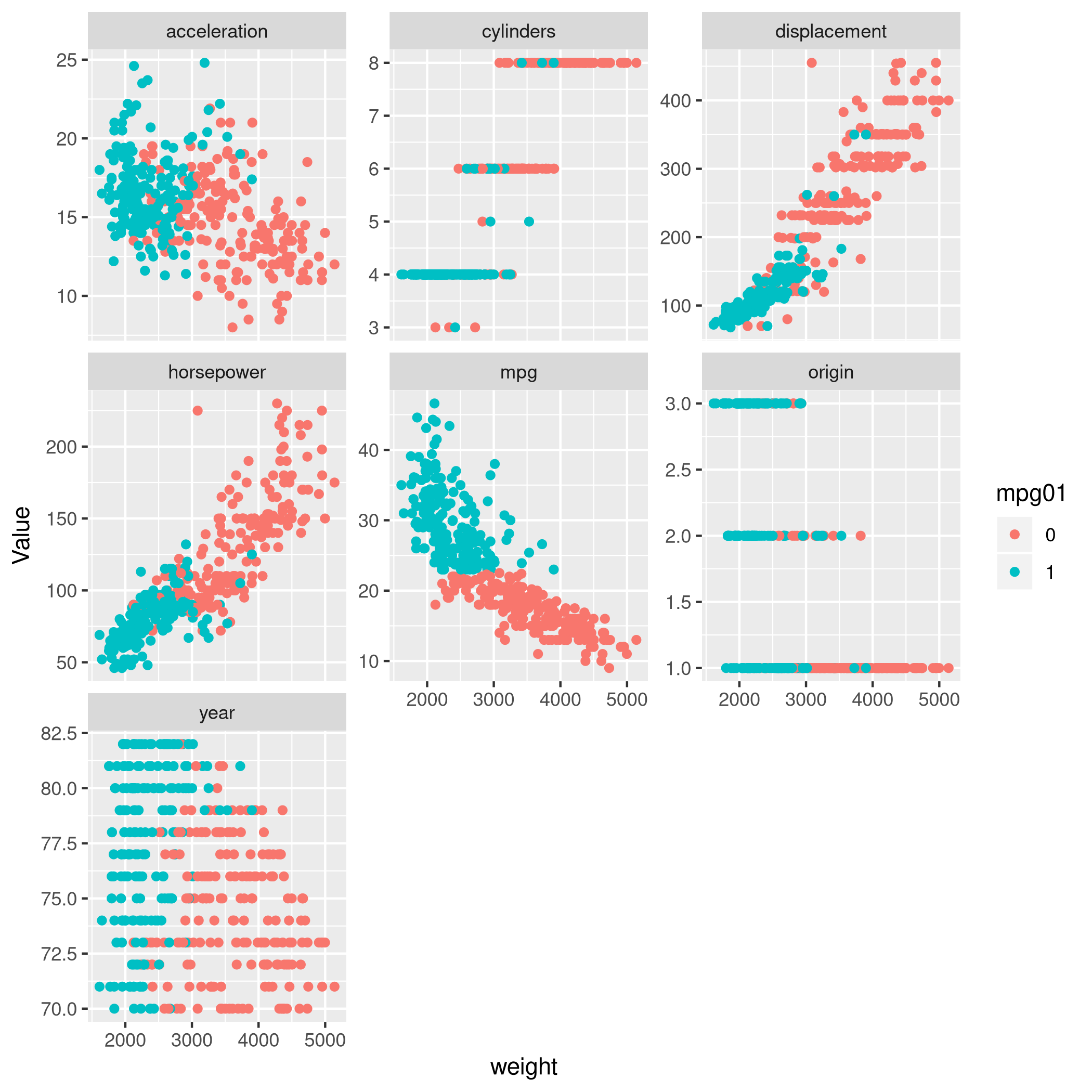Figure 13: Scatter plots Clearly, origin, year and cylinder are essentially not very relevant numerically for the regression lines and confidence intervals. newDat %>% select(-year,-origin,-cylinders) %>% pivot_longer(-c(mpg01,name,mpg),names_to="Params",values_to="Value") %>% ggplot(aes(x=mpg,y=Value,color=mpg01)) + geom_point() + geom_smooth(method=lm) + facet_wrap(~ Params, scales = "free_y")  ### c) Train-Test Split We can split our data very easily with caret. It is important to remember that for factors, random sampling occurs within each class to preserve the overall class distribution of the data. set.seed(1984) trainInd <- createDataPartition(newDat$mpg01, # Factor, so class sampling
p=0.7, # 70-30 train-test
list=FALSE, # No lists
times=1) # No bootstrap
autoTrain<-newDat[trainInd,]
autoTest<-newDat[-trainInd,]


### d) LDA with Significant Variables

Whenever I see significant I think correlation, so let’s take a look at that.

newDat %>% select(-mpg01,-name) %>% cor

#                     mpg  cylinders displacement horsepower     weight
# mpg           1.0000000 -0.7776175   -0.8051269 -0.7784268 -0.8322442
# cylinders    -0.7776175  1.0000000    0.9508233  0.8429834  0.8975273
# displacement -0.8051269  0.9508233    1.0000000  0.8972570  0.9329944
# horsepower   -0.7784268  0.8429834    0.8972570  1.0000000  0.8645377
# weight       -0.8322442  0.8975273    0.9329944  0.8645377  1.0000000
# acceleration  0.4233285 -0.5046834   -0.5438005 -0.6891955 -0.4168392
# year          0.5805410 -0.3456474   -0.3698552 -0.4163615 -0.3091199
# origin        0.5652088 -0.5689316   -0.6145351 -0.4551715 -0.5850054
#              acceleration       year     origin
# mpg             0.4233285  0.5805410  0.5652088
# cylinders      -0.5046834 -0.3456474 -0.5689316
# displacement   -0.5438005 -0.3698552 -0.6145351
# horsepower     -0.6891955 -0.4163615 -0.4551715
# weight         -0.4168392 -0.3091199 -0.5850054
# acceleration    1.0000000  0.2903161  0.2127458
# year            0.2903161  1.0000000  0.1815277
# origin          0.2127458  0.1815277  1.0000000

newDat %>% length

#  10


Now lets quickly see what it looks like with correlated values removed.

corrCols2=newDat %>% select(-mpg01,-name) %>% cor %>% findCorrelation(cutoff=0.85)
newRed<-newDat[-c(corrCols2)]
newRed %>% summary

#       mpg            weight      acceleration        year           origin
#  Min.   : 9.00   Min.   :1613   Min.   : 8.00   Min.   :70.00   Min.   :1.000
#  1st Qu.:17.00   1st Qu.:2225   1st Qu.:13.78   1st Qu.:73.00   1st Qu.:1.000
#  Median :22.75   Median :2804   Median :15.50   Median :76.00   Median :1.000
#  Mean   :23.45   Mean   :2978   Mean   :15.54   Mean   :75.98   Mean   :1.577
#  3rd Qu.:29.00   3rd Qu.:3615   3rd Qu.:17.02   3rd Qu.:79.00   3rd Qu.:2.000
#  Max.   :46.60   Max.   :5140   Max.   :24.80   Max.   :82.00   Max.   :3.000
#
#                  name     mpg01
#  amc matador       :  5   0:196
#  ford pinto        :  5   1:196
#  toyota corolla    :  5
#  amc gremlin       :  4
#  amc hornet        :  4
#  chevrolet chevette:  4
#  (Other)           :365


Inherent in this discussion is the fact that I consider what is correlated to mpg to be a good indicator of what will help mpg01 for obvious reasons.

Now we can just use the columns we found with findCorrelation.

corrCols2 %>% print

#  3 4 2

names(newDat)

#   "mpg"          "cylinders"    "displacement" "horsepower"   "weight"
#   "acceleration" "year"         "origin"       "name"         "mpg01"

autoLDA=train(mpg01~cylinders+displacement+horsepower,data=autoTrain,method="lda")
valScoreLDA=predict(autoLDA,autoTest)


Now we can check the statistics.

confusionMatrix(valScoreLDA,autoTest$mpg01)  # Confusion Matrix and Statistics # # Reference # Prediction 0 1 # 0 56 2 # 1 2 56 # # Accuracy : 0.9655 # 95% CI : (0.9141, 0.9905) # No Information Rate : 0.5 # P-Value [Acc > NIR] : <2e-16 # # Kappa : 0.931 # # Mcnemar's Test P-Value : 1 # # Sensitivity : 0.9655 # Specificity : 0.9655 # Pos Pred Value : 0.9655 # Neg Pred Value : 0.9655 # Prevalence : 0.5000 # Detection Rate : 0.4828 # Detection Prevalence : 0.5000 # Balanced Accuracy : 0.9655 # # 'Positive' Class : 0 #  That is an amazingly accurate model. auto_ldaROC<-roc(predictor=as.numeric(valScoreLDA),response=autoTest$mpg01,levels=levels(autoTest$mpg01))  # Setting direction: controls < cases  ggroc(auto_ldaROC)  ### e) QDA with Significant Variables Same deal as before. autoQDA=train(mpg01~cylinders+displacement+horsepower,data=autoTrain,method="qda") valScoreQDA=predict(autoQDA,autoTest)  Now we can check the statistics. confusionMatrix(valScoreQDA,autoTest$mpg01)

# Confusion Matrix and Statistics
#
#           Reference
# Prediction  0  1
#          0 56  2
#          1  2 56
#
#                Accuracy : 0.9655
#                  95% CI : (0.9141, 0.9905)
#     No Information Rate : 0.5
#     P-Value [Acc > NIR] : <2e-16
#
#                   Kappa : 0.931
#
#  Mcnemar's Test P-Value : 1
#
#             Sensitivity : 0.9655
#             Specificity : 0.9655
#          Pos Pred Value : 0.9655
#          Neg Pred Value : 0.9655
#              Prevalence : 0.5000
#          Detection Rate : 0.4828
#    Detection Prevalence : 0.5000
#       Balanced Accuracy : 0.9655
#
#        'Positive' Class : 0
#

auto_qdaROC<-roc(predictor=as.numeric(valScoreQDA),response=autoTest$mpg01,levels=levels(autoTest$mpg01))

# Setting direction: controls < cases

ggroc(auto_qdaROC)


OK, this is weird enough to check if it isn’t some sort of artifact.

autoQDA2=train(mpg01~horsepower, data=autoTrain,method='qda')
valScoreQDA2=predict(autoQDA2, autoTest)
confusionMatrix(valScoreQDA2,autoTest$mpg01)  # Confusion Matrix and Statistics # # Reference # Prediction 0 1 # 0 42 3 # 1 16 55 # # Accuracy : 0.8362 # 95% CI : (0.7561, 0.8984) # No Information Rate : 0.5 # P-Value [Acc > NIR] : 4.315e-14 # # Kappa : 0.6724 # # Mcnemar's Test P-Value : 0.005905 # # Sensitivity : 0.7241 # Specificity : 0.9483 # Pos Pred Value : 0.9333 # Neg Pred Value : 0.7746 # Prevalence : 0.5000 # Detection Rate : 0.3621 # Detection Prevalence : 0.3879 # Balanced Accuracy : 0.8362 # # 'Positive' Class : 0 #  OK, so the model isn’t completely creepily correct all the time. In this case we should probably think about what is going on. I would think it is because of the nature of the train-test split we performed. We have ensured during the sampling of our data that the train and test sets contain the SAME distribution (assumed). So that’s why our training result and test results are both incredibly good. They’re essentially the same thing. In fact, this is the perfect time to consider a validation set, just to see what the models are really doing. Won’t get into it right now though. ### f) Logistic with Significant Variables glmAuto.fit=glm(mpg01~cylinders+displacement+horsepower, data=autoTrain, family=binomial)  glmAuto.probs = predict(glmAuto.fit,autoTest, type = "response") glmAuto.pred = rep(1,length(glmAuto.probs)) glmAuto.pred[glmAuto.probs<0.5]=0 glmAuto.pred=factor(glmAuto.pred) confusionMatrix(glmAuto.pred,autoTest$mpg01)

# Confusion Matrix and Statistics
#
#           Reference
# Prediction  0  1
#          0 56  4
#          1  2 54
#
#                Accuracy : 0.9483
#                  95% CI : (0.8908, 0.9808)
#     No Information Rate : 0.5
#     P-Value [Acc > NIR] : <2e-16
#
#                   Kappa : 0.8966
#
#  Mcnemar's Test P-Value : 0.6831
#
#             Sensitivity : 0.9655
#             Specificity : 0.9310
#          Pos Pred Value : 0.9333
#          Neg Pred Value : 0.9643
#              Prevalence : 0.5000
#          Detection Rate : 0.4828
#    Detection Prevalence : 0.5172
#       Balanced Accuracy : 0.9483
#
#        'Positive' Class : 0
#


### g) KNN Modeling

Scale the parameters later.

knnAuto=train(mpg01~cylinders+displacement+horsepower,data=autoTrain,method="knn")


Plot KNN with the best parameters.

plot(knnCaret)


Evidently, the accuracy increases with an increase in the number of neighbors considered.

plot(knnAuto, print.thres = 0.5, type="S")


So we can see that $$5$$ neighbors is a good compromise.

## Question 4.12 - Pages 172-173

This problem involves writing functions.

(a) Write a function, Power() , that prints out the result of raising 2 to the 3rd power. In other words, your function should compute 2^3 and print out the results.

Hint: Recall that x^a raises x to the power a. Use the print() function to output the result.

(b) Create a new function, Power2() , that allows you to pass any two numbers, x and a , and prints out the value of x^a . You can do this by beginning your function with the line

Power2=function(x,a){}


You should be able to call your function by entering, for instance,

Power2(3,8)


on the command line. This should output the value of $$3^8$$, namely, $$6,651$$.

(c) Using the Power2() function that you just wrote, compute $$10^3$$, $$8^{17}$$, and $$131^3$$.

(d) Now create a new function, Power3(), that actually returns the result x^a as an R object, rather than simply printing it to the screen. That is, if you store the value x^a in an object called result within your function, then you can simply return() this result, using the following line:

return(result)


The line above should be the last line in your function, before the } symbol.

(e) Now using the Power3() function, create a plot of $$f(x)=x^2$$. The x-axis should display a range of integers from $$1$$ to $$10$$, and the y-axis should display $$x^2$$ . Label the axes appropriately, and use an appropriate title for the figure. Consider displaying either the x-axis, the y-axis, or both on the log-scale. You can do this by using log=‘‘x’’, log=‘‘y’’, or log=‘‘xy’’ as arguments to the plot() function.

(f) Create a function, PlotPower() , that allows you to create a plot of x against x^a for a fixed a and for a range of values of x. For instance, if you call

PlotPower (1:10 ,3)


then a plot should be created with an x-axis taking on values $$1,2,…,10$$ and a y-axis taking on values $$1^3,2^3,…,10^3$$

### a) Create a Squaring Function

Power=function(x){print(2^x)}
Power(3)

#  8


### b) Generalizing Power to arbitrary numbers

Power2=function(x,a){print(x^a)}

Power2(3,8)

#  6561


### c) Random Testing of Power2

Power2(10,3)

#  1000

Power2(8,17)

#  2.2518e+15

Power2(131,2)

#  17161


### d) Return a value

Power3=function(x,a){return(x^a)}


### e) Plot something with Power3

Actually now would be a good place to introduce LaTeX labeling.

#install.packages("latex2exp")
library(latex2exp)


No log scale.

qplot(x=seq(1,10),y=Power3(seq(1,10),2)) + ggtitle("Function without a log scale") +
geom_point() + xlab("X") + ylab(TeX("$X^2$"))


With a log scale.

qplot(x=seq(1,10),y=Power3(seq(1,10),2)) + ggtitle("Function with a log scale") +
geom_point() + xlab("X") + ylab(TeX("$X^2$")) + scale_y_log10()


### f) PlotPower Function

PlotPower=function(xrange,pow){return(qplot(x=xrange,y=Power3(xrange,pow)))}

plotter<-PlotPower(1:10,3)
plotter


The R Cookbook is quite neat for some simple tasks like this.

## Question 4.13 - Pages 173

Using the Boston data set, fit classification models in order to predict whether a given suburb has a crime rate above or below the median. Explore logistic regression, LDA, and KNN models using various subsets of the predictors. Describe your findings.

OK, to speed this up, I will simply run through all the work done on the Auto set. Recall that details about this data-set are also here.

boston<-MASS::Boston

• Check unique values
boston %>% sapply(unique) %>% sapply(length)

#    crim      zn   indus    chas     nox      rm     age     dis     rad     tax
#     504      26      76       2      81     446     356     412       9      66
# ptratio   black   lstat    medv
#      46     357     455     229


CHAS is of course something which should be a factor, and with RAD having only $$9$$ levels, I’m inclined to make it a factor as well.

boston<-boston %>% mutate(rad=factor(rad),chas=factor(chas))

• Make a median variable
boston$highCrime<- ifelse(boston$crim<boston$crim %>% median(),0,1) %>% factor()  • Take a look at the data Some box-plots: boston %>% pivot_longer(-c(rad,chas,highCrime),names_to="Param",values_to="Value") %>% ggplot(aes(x=highCrime,y=Value,fill=chas)) + geom_boxplot()+ facet_wrap(~Param,scales="free_y")  It is surprising, but evidently the CHAS variable is strangely relevant. 1 implies the tract bounds the river, otherwise 0. • Correlations boston %>% select(-c(rad,chas,highCrime)) %>% cor  # crim zn indus nox rm age # crim 1.0000000 -0.2004692 0.4065834 0.4209717 -0.2192467 0.3527343 # zn -0.2004692 1.0000000 -0.5338282 -0.5166037 0.3119906 -0.5695373 # indus 0.4065834 -0.5338282 1.0000000 0.7636514 -0.3916759 0.6447785 # nox 0.4209717 -0.5166037 0.7636514 1.0000000 -0.3021882 0.7314701 # rm -0.2192467 0.3119906 -0.3916759 -0.3021882 1.0000000 -0.2402649 # age 0.3527343 -0.5695373 0.6447785 0.7314701 -0.2402649 1.0000000 # dis -0.3796701 0.6644082 -0.7080270 -0.7692301 0.2052462 -0.7478805 # tax 0.5827643 -0.3145633 0.7207602 0.6680232 -0.2920478 0.5064556 # ptratio 0.2899456 -0.3916785 0.3832476 0.1889327 -0.3555015 0.2615150 # black -0.3850639 0.1755203 -0.3569765 -0.3800506 0.1280686 -0.2735340 # lstat 0.4556215 -0.4129946 0.6037997 0.5908789 -0.6138083 0.6023385 # medv -0.3883046 0.3604453 -0.4837252 -0.4273208 0.6953599 -0.3769546 # dis tax ptratio black lstat medv # crim -0.3796701 0.5827643 0.2899456 -0.3850639 0.4556215 -0.3883046 # zn 0.6644082 -0.3145633 -0.3916785 0.1755203 -0.4129946 0.3604453 # indus -0.7080270 0.7207602 0.3832476 -0.3569765 0.6037997 -0.4837252 # nox -0.7692301 0.6680232 0.1889327 -0.3800506 0.5908789 -0.4273208 # rm 0.2052462 -0.2920478 -0.3555015 0.1280686 -0.6138083 0.6953599 # age -0.7478805 0.5064556 0.2615150 -0.2735340 0.6023385 -0.3769546 # dis 1.0000000 -0.5344316 -0.2324705 0.2915117 -0.4969958 0.2499287 # tax -0.5344316 1.0000000 0.4608530 -0.4418080 0.5439934 -0.4685359 # ptratio -0.2324705 0.4608530 1.0000000 -0.1773833 0.3740443 -0.5077867 # black 0.2915117 -0.4418080 -0.1773833 1.0000000 -0.3660869 0.3334608 # lstat -0.4969958 0.5439934 0.3740443 -0.3660869 1.0000000 -0.7376627 # medv 0.2499287 -0.4685359 -0.5077867 0.3334608 -0.7376627 1.0000000  Now, unsurprisingly, there’s nothing which is really strongly correlated here for some reason. • Train test splits set.seed(1984) trainIndCri <- createDataPartition(boston$highCrime, # Factor, so class sampling
p=0.7, # 70-30 train-test
list=FALSE, # No lists
times=1) # No bootstrap
bostonTrain<-boston[trainIndCri,]
bostonTest<-boston[-trainIndCri,]

• Make a bunch of models
glmBos.fit=glm(highCrime~., data=bostonTrain, family=binomial)

# Warning: glm.fit: algorithm did not converge

# Warning: glm.fit: fitted probabilities numerically 0 or 1 occurred

glmBos.probs = predict(glmBos.fit,bostonTest, type = "response")
glmBos.pred = rep(1,length(glmBos.probs))
glmBos.pred[glmBos.probs<0.5]=0
glmBos.pred=factor(glmBos.pred)
confusionMatrix(glmBos.pred,bostonTest$highCrime)  # Confusion Matrix and Statistics # # Reference # Prediction 0 1 # 0 68 6 # 1 7 69 # # Accuracy : 0.9133 # 95% CI : (0.8564, 0.953) # No Information Rate : 0.5 # P-Value [Acc > NIR] : <2e-16 # # Kappa : 0.8267 # # Mcnemar's Test P-Value : 1 # # Sensitivity : 0.9067 # Specificity : 0.9200 # Pos Pred Value : 0.9189 # Neg Pred Value : 0.9079 # Prevalence : 0.5000 # Detection Rate : 0.4533 # Detection Prevalence : 0.4933 # Balanced Accuracy : 0.9133 # # 'Positive' Class : 0 #  bostonLDA=train(highCrime~.,data=bostonTrain,method='lda') bostonQDA=train(highCrime~tax+crim,data=bostonTrain,method='qda') bostonKNN=train(highCrime~.,data=bostonTrain,preProcess = c("center","scale"),method='knn')  bLDAp=predict(bostonLDA,bostonTest) bQDAp=predict(bostonQDA,bostonTest) bKNNp=predict(bostonKNN,bostonTest)  confusionMatrix(bLDAp,bostonTest$highCrime)

# Confusion Matrix and Statistics
#
#           Reference
# Prediction  0  1
#          0 72  6
#          1  3 69
#
#                Accuracy : 0.94
#                  95% CI : (0.8892, 0.9722)
#     No Information Rate : 0.5
#     P-Value [Acc > NIR] : <2e-16
#
#                   Kappa : 0.88
#
#  Mcnemar's Test P-Value : 0.505
#
#             Sensitivity : 0.9600
#             Specificity : 0.9200
#          Pos Pred Value : 0.9231
#          Neg Pred Value : 0.9583
#              Prevalence : 0.5000
#          Detection Rate : 0.4800
#    Detection Prevalence : 0.5200
#       Balanced Accuracy : 0.9400
#
#        'Positive' Class : 0
#

confusionMatrix(bQDAp,bostonTest$highCrime)  # Confusion Matrix and Statistics # # Reference # Prediction 0 1 # 0 73 5 # 1 2 70 # # Accuracy : 0.9533 # 95% CI : (0.9062, 0.981) # No Information Rate : 0.5 # P-Value [Acc > NIR] : <2e-16 # # Kappa : 0.9067 # # Mcnemar's Test P-Value : 0.4497 # # Sensitivity : 0.9733 # Specificity : 0.9333 # Pos Pred Value : 0.9359 # Neg Pred Value : 0.9722 # Prevalence : 0.5000 # Detection Rate : 0.4867 # Detection Prevalence : 0.5200 # Balanced Accuracy : 0.9533 # # 'Positive' Class : 0 #  confusionMatrix(bKNNp,bostonTest$highCrime)

# Confusion Matrix and Statistics
#
#           Reference
# Prediction  0  1
#          0 74  6
#          1  1 69
#
#                Accuracy : 0.9533
#                  95% CI : (0.9062, 0.981)
#     No Information Rate : 0.5
#     P-Value [Acc > NIR] : <2e-16
#
#                   Kappa : 0.9067
#
#  Mcnemar's Test P-Value : 0.1306
#
#             Sensitivity : 0.9867
#             Specificity : 0.9200
#          Pos Pred Value : 0.9250
#          Neg Pred Value : 0.9857
#              Prevalence : 0.5000
#          Detection Rate : 0.4933
#    Detection Prevalence : 0.5333
#       Balanced Accuracy : 0.9533
#
#        'Positive' Class : 0
#


Clearly in this particular case, an LDA model seems to be working out the best for this data when trained on all the parameters, though Logistic Regression is doing quite well too.

• Notes on KNN
plot(bostonKNN)

plot(bostonKNN, print.thres = 0.5, type="S")

• Comparison

Finally, we will quickly plot some indicative measures.

knnBosROC<-roc(predictor=as.numeric(bKNNp),response=bostonTest$highCrime)  # Setting levels: control = 0, case = 1  # Setting direction: controls < cases  logiBosROC<-roc(predictor=as.numeric(glmBos.probs),response=bostonTest$highCrime)

# Setting levels: control = 0, case = 1
# Setting direction: controls < cases

ldaBosROC<-roc(predictor=as.numeric(bLDAp),response=bostonTest$highCrime)  # Setting levels: control = 0, case = 1 # Setting direction: controls < cases  qdaBosROC<-roc(predictor=as.numeric(bQDAp),response=bostonTest$highCrime)

# Setting levels: control = 0, case = 1
# Setting direction: controls < cases

ggroc(list(KNN=knnBosROC,Logistic=logiBosROC,LDA=ldaBosROC,QDA=qdaBosROC))

OK, one of the reasons why these models do so well is because they are all assuming an equal distribution of train and test classes, and they use crim itself as a predictor. This is no doubt a strong reason why these models uniformly perform so well. I’d say 5 is the best option.